# Using DATE function in Excel - formula examples to calculate dates

When it comes to calculating dates in Excel, DATE is the most essential function to understand. As you probably know, Excel does not keep the year, month and day for a date, nor does it explicitly store weekday information in a cell. Instead, Microsoft Excel stores dates as serial numbers and this is the main source of confusion.

Not all Excel date functions can recognize dates entered as text values, therefore it's not recommended to supply dates directly in calculations. Instead, you should use the DATE function to get a serial number representing the date, the number that Excel understands and can operate on.

## Excel DATE function

The Excel DATE function returns the serial number that represents a certain date. It has the following arguments:

DATE(year, month, day)

Where:

Year - represents the year of the date.

Month - an integer representing the month of the year, from 1 (January) to 12 (December).

Day - an integer corresponding to the day of the month, from 1 to 31.

The DATE function is available in all versions of Excel 365 - Excel 2007.

### DATE function - tips and notes

The DATE syntax looks crystal clear and straightforward on the surface. In practice, there may be some unobvious pitfalls that the below tips will help you avoid.

#### Year

Excel interprets the year argument according to the date system set up on your computer. By default, Microsoft Excel for Windows uses the 1900 system where January 1, 1900 is represented by the serial number 1. For more details, please see Excel date format.

• If the year argument is between 1900 and 9999 inclusive, Excel uses exactly the value you supplied to create a date. For example, DATE(2015, 12, 31) returns December 31, 2015.
• If the year argument is between 0 and 1899 inclusive, Excel adds the specified number to 1900. For example, DATE(100, 12, 31) returns December 31, 2000 (1900 + 100).
• If the year argument is less than 0 or greater than 9999, a DATE formula will return the #NUM! error.

Tip. To avoid confusion, always supply four-digit years. For example, if you input "01" or "1" in the year argument, your DATE formula will return the year of 1901.

#### Month

• If the month argument is greater than 12, Excel adds that number to the first month in the specified year. For example, DATE(2015, 15, 5) returns the serial number representing March 1, 2016 (January 5, 2015 plus 15 months).
• If the month argument is less than 1 (zero or negative value), Excel subtracts the magnitude of that number of months, plus 1, from the first month in the specified year. For example, DATE(2015, -5, 1) returns the serial number representing July 1, 2014 (January 1, 2015 minus 6 months).

#### Day

As well as month, the day argument can be supplied as a positive and negative number, and Excel calculates its value based on the same principles as described above.

Tip. At first sight, supplying negative values in the month or day argument of the Excel DATE function may seem absurd, but in practice it may turn out quite useful, for example in the complex formula that converts a week number to a date.

## Excel DATE formula examples

Below you will find a few examples of using DATE formulas in Excel beginning with the simplest ones.

### Example 1. DATE formula to return a serial number for a date

This is the most obvious use of the DATE function in Excel.

For example, to return a serial number corresponding to 20-May-2015, use this formula:

`=DATE(2015, 5, 20)`

Instead of specifying the values representing the year, month and day directly in a formula, you can have some or all arguments driven by of other Excel date functions. For instance, combine the YEAR and TODAY to get a serial number for the first day of the current year.

`=DATE(YEAR(TODAY()), 1, 1)`

And this formula outputs a serial number for the first day of the current month in the current year:

`=DATE(YEAR(TODAY()), MONTH(TODAY(), 1)`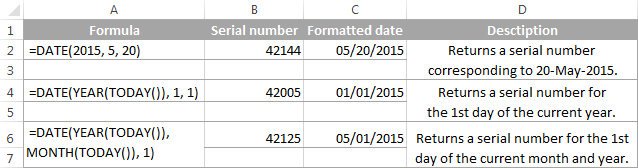Tip. To display a date rather than a serial number, apply the desired Date format to the formula cell.

### Example 2. How to return a date based on values in other cells

The DATE function is very helpful for calculating dates where the year, month, and day values are stored in other cells.

For example, to find the serial number for the date, taking the values in cells A2, A3 and A4 as the year, month and day arguments, respectively, the formula is:

`=DATE(A2, A3, A4)`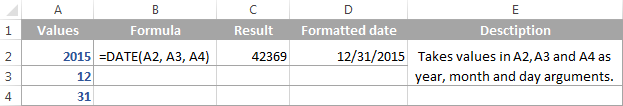### Example 3. DATE formula to convert a string or number to a date

Another scenario when the Excel DATE function proves useful is when the dates are stored in the format that Microsoft Excel does not recognize, for instance DDMMYYYY. In this case, you can use DATE in liaison with other functions to convert a date stored as a numeric string or number into a date:

`=DATE(RIGHT(A2,4), MID(A2,3,2), LEFT(A2,2))`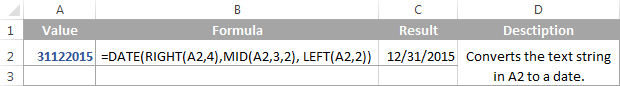### Example 4. Adding and subtracting dates in Excel

As already mentioned, Microsoft Excel stores dates as serial numbers and operates on those numbers in formulas and calculations. That is why when you want to add or subtract some days to/from a given date, you need to convert that date to a serial number first by using the Excel DATE function. For example:

• Adding days to a date:`=DATE(2015, 5, 20) + 15`The formula adds 15 days to May 20, 2015 and returns June 4, 2015.
• Subtracting days from a date:`=DATE(2015, 5, 20) - 15`The result of the above formula is May 5, 2015, which is May 20, 2015 minus 15 days.
• To subtract a date from today's date:`=TODAY()-DATE(2015,5,20)`The formula calculates how many days are between the current date and some other date that you specify.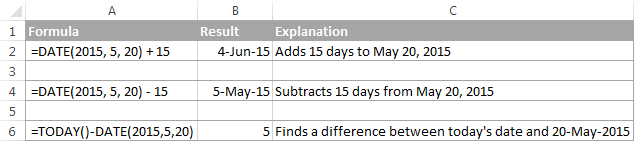If you are adding or subtracting two dates that are stored in some cells, then the formula is as simple as =A1+B1 or A1-B1, respectively.

And here are a few more examples where Excel DATE is used in combination with other functions in more complex formulas:

## Using Excel DATE formulas in conditional formatting

In case you want not only to calculate but also highlight dates in your Excel worksheets, then create conditional formatting rules based on DATE formulas.

Supposing you have a list of dates in column A and you want to shade dates that occurred earlier than 1-May-2015 in orange and those that occur after 31-May-2015 in green.

The DATE formulas you want are as follows:

Orange: `=\$A2<DATE(2015, 5, 1)` - highlights dates less than 1-May-2015

Green: `=\$A2>DATE(2015, 5, 31)` - highlights dates greater than 31-May-2015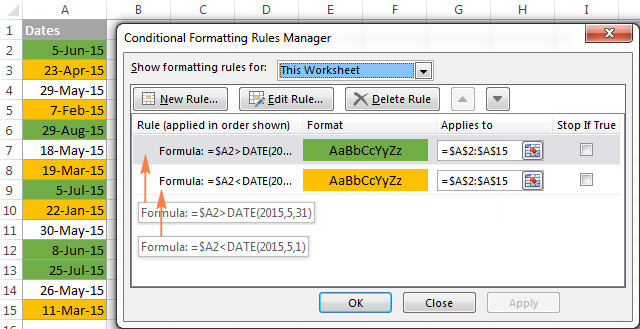For the detailed steps and more formula examples, please see How to conditionally format dates in Excel.

## How to make date formulas in Excel with Date & Time Wizard

Though DATE is the main function to work with dates in Excel, a handful of other functions are available to tackle more specific tasks. You can find the links to in-depth tutorials at the end of this article.

Meanwhile, I'd like to present you our Date & Time Wizard - a quick and easy way to calculate dates in Excel. The beauty of this tool is that outputs the results as formulas, not values. Thus you have a kind of 'two birds, one stone' opportunity - get the result faster and learn Excel date functions along the way :)

The wizard can perform the following calculations:

• Add years, months, weeks, days, hours, minutes and seconds to the specified date.
• Subtract years, months, weeks, days, hours, minutes and seconds from the specified date.
• Calculate the difference between two dates.
• Get age from the birthdate.

For example, here's how you can add 4 different units in cells B3:E3 to the date in A4. The formula in B4 is built in real-time as you change the conditions: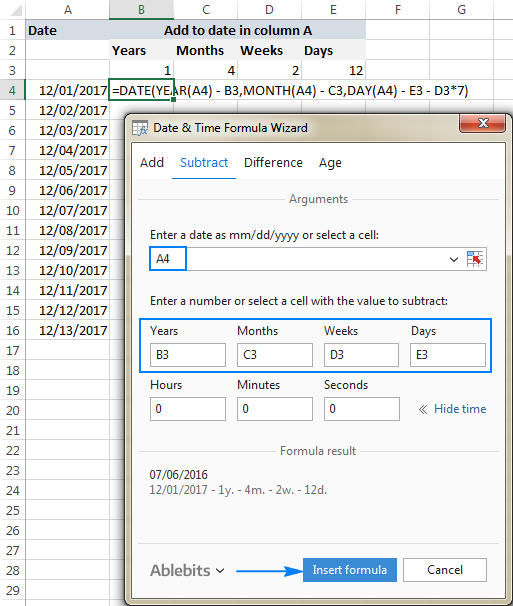If you are curious to explore other capabilities of the wizard, feel free to download an evaluation version of the Ultimate Suite below which includes this as well as 60 more time saving add-ins for Excel.

I thank you for reading and hope to see you on our blog next week!

## Excel dates tutorials:

1. I'm ddoing a spreadsheet for my budget of the different bills I owe. My issue is that when I enter new dates for next year it adds 2016 instead of 2017. Can someone tell me how to fix this? I should be able to tpe in the month/date hit enter and the year auto populate but it's doing it for 2016.

TIA

2. Thank you for all your wonderful knowledge. Would you be able to help me do the following?

I need to have a specific starting date such as 1/1/17. I need the formula to calculate 91 days into the future that is a Wednesday closest to the 91st day but not less than the 91 days. The starting date will change every time I need to make an entry, so the formula will calculate the best Wednesday.
thank you,
Brian

3. I am trying to calculate the difference between starting and ending time on a work shift. The calculation is okay if the hours are all in the same day. i.e. start 8:00 am and quit at 5:00 pm.

The problem I am having is if the starting time is 6:00 pm and the ending time is 3:00 am. I was hoping the answer would be 9 hours. Instead, the answer is 15 hours.

The cells are formatted in hh:mm. The cells are not formatted like dd-mm-yyyy hh:mm because of the number of employees that need to be entered.

4. Hello, I am a newbie at this and trying to wing it the best i can to get a jump start. I am trying essentially to calculate 30 day, 60 day, and 90 day increments based on a random start day to arrive at an end day for late fees. I have to of course consider 30 and 31 day months and february of course being a 28 day month this year. Holidays are not an issue. Can you help me figure out how to enter this formula in excell? Thank you!

5. Sorry I can't find a solution to what I'm looking for in the large amounts of questions. Any help greatly appreciated:

I'd like a formula that adds a certain value, or indeed a multiplier, based on a date of the month. i.e a cell that adds another €100 automatically every 25th of the month, even better if it can stop after a year (Jan-Dec)

I'm aware there would be the very long IF function variant using TODAY and a bit of juggling, but there must be a neater formula?

Would love to hear some ideas. Thanks!

• A botched workaround to my above question:

=IF(TODAY()<DATE(2016,12,31),(ROUND(((TODAY()-DATE(2016,1,1))/30),0)*[desired value]),(12*[desired value]))

Does the trick, just only comes into effect half way through the month and assumes 30 days in each month.

Would love to hear how to nail it down to a specific date.

6. What formula to use if you wants to know age calculation.
E.g. born on 15th May 2010 and I want to auto calculate age to the date of 31st Dec 2020 ?

7. I have a spreadsheet broken down by pay periods and want to apply a formula to change the range of week dates in each subsequent cell. Example: Pay period 1 in January 2017 is 8-14 for the first week and 15-21 for the second. I want a formula to deliver 22-28 in the next cell so I don't have to look at a calendar and manually enter the dates.

8. Hi,

What about payroll cut-off date to be published in a cell, say for example cut-off date is from January 1 to 15, 2016. Is there a formula to show this in a single excel cell? Thanks in advance.

9. Hi,

The answer to the above question (# 141) is
=IF((AND(E2"",F2"")),(MIN(TODAY(),F2)-E2+1)/(F2-E2+1),"")

10. Hi,

I have to calculate percentage between two dates and the formula I'm using is =(MIN(TODAY(),F2)-E2+1)/(F2-E2+1) which is giving me the desired result. However, when I do not enter any dates in E2 and F2, I get a default result of 4272000% in cell G2.

I want G2 to be blank when no information is available in E2 and F2, Also it should be blank if I enter dates in one of the cells E2 or F2 or even postdates.

I would really appreciate if a formula could be devised in coordination with the above percentage formula to achieve the desired results.

11. I have a start date in A1 and End date in B1 of Construction project, I want first the formula calculate the number of days overdue with today date, and then check if the end date is greater than today then return "Not Due" otherwise calculate number of days overdue.

12. I wondered if anyone could advise how to add fifteen days to a date and if more than return 'no' in another column with a count of how many days? Similarly with anything less than so for example:

Greater than:
Col A
01/01/16

Column b
17/01/16

Column C
'No - 1 day'

Less than:
Col A
01/01/16

Column b
15/01/16

Column C
'Yes'

13. Great site! I am need of help with a specific date formula. It is as follows:

I have a specific date that is calculated based on lead times in manufacturing. From that, I have a plant actual ship day of the week. Starting with the specific date, I need to calculate the next plant shipdate as an actual date, not day of week. Is there a formula for doing this?

Example:
Specific Date: 12/21/2016
Plant Ship Day: Tuesday (depending on plant, this can be any day of the week so I have created a drop with all 7 weekdays to chose from)
Needed: Next available ship date. In this example, 12/21/2016 is a Wednesday so the formula to determine the next Tuesday should equal 12/27/2016.

14. Hi All,

I need a formula for create a list of sequential dates.

I have 2 slicer"Month" and "year" and holiday list also. if i select particular month and year from slicer, i need the end to end dates without holiday list

For example: If select 2016 and Feb, I need dates without holiday list in column a1 2/1/2016 a2- 2/2/2016 a3- 2/3/2016------ last column 2/29/2016

15. Im need a formula thta will add 6 month to a date in in cell A2 but if there is no date to return a zero

16. Hi,

I'm trying to get number of days between two dates. There are a number of ways to do it, but I'm not able to find one to suit my purpose to calculate vacation days!

Eg: Cell A2 dates 01/12/2016 and cell B2 10/12/2016. So the person is on leave from Dec 1 to Dec. 10 which is 10 calendar days, but all the formulas I know show it as 9 days.

Thank you

17. Hi,

How to calculate specific date in the next 5th years from a specific date or today's date?

Regards,
Santosh

18. Hello,
I want to audit if a form was present by the 30th day and by the 90th day. I want a clean spreadsheet to use as a template every month. When I use =B3+30 in one cell and =B3+90 in another cell it returns 1/30/00 since B3 is blank. I want this as my template every month and I would like the formula cell empty until B3 has a value.

Thank you!

19. 21/nov/2016 how it will be done with date function or any other function.

20. Hello ,

I am trying to develop a spreadsheet that I can input the date and automatically generate the date of the 6 month review ... is that possible ?

21. Hello, I would like to have an automatique date put in when i put a X in a colume. But I do not want that date to change once it is put it in. I tried this but once we change date the date in the colume changes to and i don't want that.

=IF(G4="x";NOW()) -- changes date the next day
=IF(G4="x";Today())-- changes date the next day

22. Dear All
I want to maintain a expense sheet which contains cash expense and credit expense but the problem is about the preparing for merge of cash and credit how i can please let me Know

23. Hi,
I had prepared a Attendance Sheet which is auto populated with P (present) till present date and A (Absent) if entered manually in another sheet named "Absent" with the following formula: =IF(E\$2TODAY(),"",IFERROR(VLOOKUP(\$A4&"-"&E\$2,Absent!\$A:\$E,5,0),"P")))).

My query is What is the formula for making a series of A (Absent) if one of an employee is absent from a given start date to given End date in sheet "Absent". Also what is the integrated formula for an employee who had retired /resigned on a specified date

24. Hi,

I am trying to use a formula to automatically calculate the length of time until the next meeting based off today's date. The dates of the monthly meetings will be stored in a separate worksheet. So far I believe something like the formula below should work?

=MIN(IF('Schedule V.1.1'!D13:D22>=TODAY(),'Schedule V.1.1'!D13:D22,""))

Any help would be greatly appreciated.

25. Hello,
I am trying to search for any date in three columns and add 2 years to the date it finds. any suggestions?

26. Hi Im trying to populate a calendar from one date.
eg if I enter the 20th of november this will create a number of dates and jobs based on formulas from the entered date
eg 20.11 start date will mean on 20.11 + 83 job A needs done and 20.11 + 123 job B will need done
Is this Possible?

27. How do i get a formula to change a date (12/25/10) to a # (dpd)

28. Good day,
Can you maybe help. I have a spreadsheet with the age of issues (issue log). I need to split the ageing of the issues into 60 days to count how many of the issues in each of the ageing falls to present it in a pie chart at the end.

How do I calculate the counts for each ageing categories?

Thank you,
Leana

• Sorry, I noticed the detail is incorrect - apologies.

It must be split between 60 days.

• Sorry, it seems like it converts my calculation....

It reads: It must be split between less than 30 days, 31-60 days and more than 60 days

• Hi Leana,

Please provide more information on how your data is organized. In particular, how should the aging of issues be calculated? Do you have a column of issue dates that should be compared with today's date?

29. Can you help me out with following problem.
I want to calculate total delay time and the total early time in the attendence sheet.as a example,our office start at 7:00AM. if some one came at after that time or early that time,I want to calculate total delay times and early times for a month.

30. If I don't have "B" then I want my result to be a "0".
How can I do it???

Example:
If I want to get the days since something is open and one of the dates is empty.

"A" Date of report (I will always have this information)
"B" Date the action is open (I will not have always this information)

my formula is

="A"-"B" = days since it has been open.

If I don't have "B" then I want my result to be a "0".
How can I do it???

• I found the answer :-)

=IF(B="", 0, A-B)

31. Hi

I need to set a formula which help me to auto calculate the expiry date
eg : start date is 6 Apr 15 and the expiry date is 2.5 years later

and i will use this formula for the other cells
do i need to put the \$ so that i can just drag it down

thanks

32. I need a formula to add a year to date if a specified field has "Y".

so if field A1 equals "y" then add a year to the date on A2.

33. Hello,

Since a week i am struggling with an Increment formula,
Im using formula which shows as
=IF(AA2=0,0,(F2)*VLOOKUP(\$AE2,ML!\$F\$26:\$G\$29,2,0))
Here: AA2 is the End of Contract date
F2 is the Basic Salary
Vlookup is the range of Category(Doctor,Admin,Nurses,Paramedical staff) which define the percentage in Increment.

Right now i have months in different coloumn
i want this formula to show only for those people whose dates will reflect on the End of Contract months.

for me this formula is effecting in all the months.

34. Hello,

I have an expiry date of 30-11-2016 I want to see on next column the date if I subtract 30 days on it e.g. next column should read like 31-10-2016.

35. Hi,

I am looking for a excel formula.
Working on incentive program for employees. I am using GDocs for tracking the employee performance.

I am having start date and end date. With the help of start and end date I want to pay the incentives every month. Once the project reaches end date next month should be "0".

Kindly let me know how to write the formula for this.

Note: All the docs are in GDocs & 2 different files.

Regards,
Sharath Babu S

36. I WANT TO FIND REMANING % FROM 100% WHEN GIVEN % IS 17.09

37. Hi I am trying to create a spreadsheet for vehicle finance showing vehicle, purchase date, purchase price, total interest, number of monthly payments, number of payments remaining, monthly payment, outstanding finance.

The bit I am struggling with is trying to create a formula for a cell to work out how many months are remaining. I know what I want it to do which is work out how many months are between the purchase date and end date using the current date if that makes sense.

38. i have a leads excel (clients) and i plan to calculate how many leads we got:
-yesterday
-today
-last7days
-this month
I have P column that have "7" standard possible answers; like the state of the lead. I need a formula for each state of the lead to count the nr of leads for the above time periods.

Thanks,
I really appreciate this answer i tried for one week to do it

39. = COUNTIFS(P2:P8,"Waiting List",I2:I8,TODAY())

tried this one and not working as well is saying 0

40. I tried like this:
=COUNTIF(I2:I11,"TODAY()") + COUNTIF(P2:P11,"=Waiting List")

but it's not taking in count the Date; just sum all of them matching "Waiting List"

Thanks,
Traian

41. i have a leads excel (clients) and i plan to calculate how many leads we got:
-yesterday
-today
-last7days
-this month
I have P column that have "7" standard possible answers; like the state of the lead. I need a formula for each state of the lead to count the nr of leads for the above time periods.

Thanks,
I really appreciate this answer i tried for one week to do it.

42. if i entered date by using formul =Today() the output i want same date -1 but if this date occurs on sunday it should be -2

43. Hi Mam please let me know if 1 serial nos receive in two different date then whose formula use in excel

44. Sum of Column values based on a particular row(person) like On the basis of specfic value like date Oct-2016 it gives us the Sum of other column which we select.

45. I have two dates in two separate column and I want to have which ever date comes due first to populate into a new column. How do i formulate that?

• Hi Ryan,

Assuming you are comparing dates in columns A and B, the following formula should work a treat:
=IF(A2

46. to get the result but without the last 6 months of this account code (supplier)

47. hi,
I want to exclude 6 months from total amount of a special account for making an aging report so I want the result over 180 days of base amount but without 6 months

48. I have cells formatted as text with a month & day present. I am trying to use the current date to add the current or next year depending on month & day. So for example today's date is 10/10/2016, and I have 2 inputs, 7/1 & 1/1. I'd like to format as 7/1/2016 & 1/1/2017. Any suggestions?

49. For the past year I have been using a formula to keep track of when my patients are due for their next visit. For example, if I visited today and they are due again in 4 weeks, I would plug in today's date and the formula gave me a date 28 days from today. The formula I was using was =SUM(column and row of today's date,number of days to next visit). So it might look something like this: =SUM(C3,28). That was working well up until a few weeks ago when all of a sudden, the projected date was coming up as a series of pound signs (#########). Has something changed with Excel?

• Never mind. My future date column was too narrow for double digit months. When I widened it, the pound signs turned into the date. :-S

• Yes, the column is to narrow

50. how to write date formula

• date formula ctrl+:
time gormula ctrl"